Browse by Topic
Related Topics

# From Pi to Puzzles

Neil Bickford calculated the first 458 million terms for the continued fraction of pi, breaking the previous record of 180 million. He used Mathematica to develop his code and verify his results—which he posted shortly after he turned 13.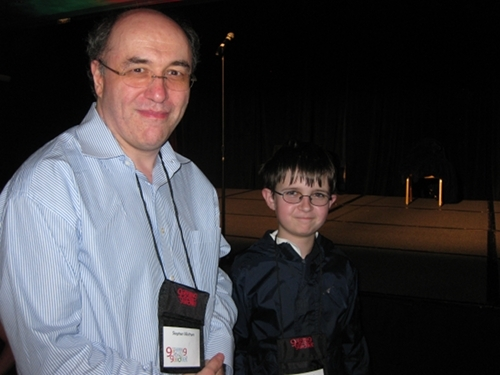Neil Bickford meets Stephen Wolfram at Gathering 4 Gardner 9.

Bickford, who broke the record last fall, said creating the early version of the pi continued-fraction generator was “the best thing I’ve ever used Mathematica for.”

On his blog Random (Blog), he wrote about the history of calculations of pi and described his computation.

A continued fraction is a way of expressing a number: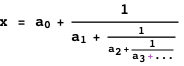It can be written more compactly as: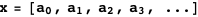Even irrational numbers might have regular continued fractions: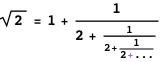Or: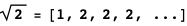The continued fraction of pi, however, does not show a pattern; it is: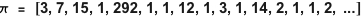After creating his code in Mathematica, Bickford used C# to develop PiCF, which computes continued fractions using an algorithm originally developed by Bill Gosper.

On his blog, Bickford wrote:

“On October 17th, I calculated approximately 458,000,000 terms of pi in about 3 hours on a 64-bit machine running Windows on a Core 2 Duo @ 3.00 GHz. This was later verified using Mathematica…. The program was coded in C#, has a command-line interface (with menus!), and uses Emil Stefanov’s wrapper of GNU MP for the BigInteger multiplications. The maximum term is still 878783625, originally found by Bill Gosper….”

Bickford said that verifying the result in Mathematica was faster than doing so in C# or another language. Plus, after using a built-in function to turn the terms back into fractions, he could check for periodicity in the fractions, which he said would have been nearly impossible any other way.

Bickford initially got into math via puzzle books by Ivan Moscovich.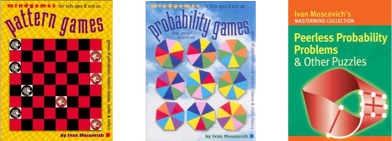When he was around 10, Bickford began using graphing software to plot and modify equations. That led him to Mathematica, which he said he liked better than other software because he could modify built-in functions easily.

“It was easier to create programs quickly in Mathematica than in other programs due to the sheer number of built-in functions,” Bickford said.

He improved his Mathematica skills by working with the “amazing number of cool examples” in the documentation.

With Mathematica, he transferred his interest from shapes to math, including math puzzles.

Recently, Bickford wrote a guest Numberplay post on The New York Times blog Wordplay in which he presented three puzzles about representing time. One of them is among his favorite puzzles. Bickford wrote the other two puzzles, and then used Mathematica to calculate the answers.

All the puzzles were about how to show different digital clock times using a limited set of rods or numbers.The third puzzle asked: If you have eight cards numbered 0 to 7, how many times can you display in the format hours:minutes:seconds? But instead of using a 24-hour day, the puzzle used the length of a day on Venus, about 243.02 Earth days. So the puzzle required figuring out how many times could be displayed up to 5832:28:47.

Bickford used Mathematica‘s Permutations function to check all the permutations by brute force. He said that if he’d tried to write a C# program, it would have taken a long time just to write the program, but Mathematica‘s built-in function sped up development.

For the answer, see his response in the comments on the Numberplay post.

For his next Mathematica project, he’s investigating sliding block puzzles, trying to find the hardest possible 4×4 puzzle. One type of 4×4 sliding block puzzle, also called the 15 puzzle, uses 15 scrambled numbered tiles. To solve the puzzle, you have to slide them one at a time into the single empty spot until they are in numerical order.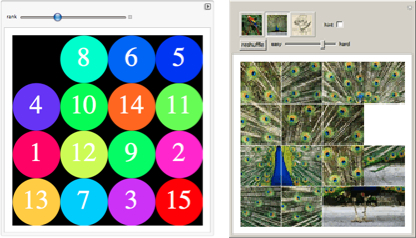See “Counting Order Puzzle” or “Picture Puzzle” in the Wolfram Demonstrations Project for examples of sliding puzzles with numbered tiles (from 3×3 up to 6×6) or with an image instead of numbers.

Bickford’s puzzles are a bit different from the 15 puzzle—he’s fused some blocks together and removed others. The goal of the puzzle below is to move the red rectangle from the upper left to the lower right.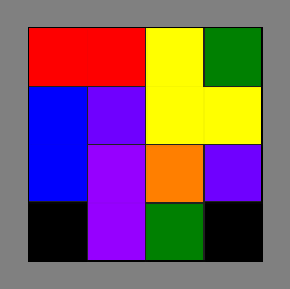The toughest puzzle he’s found so far—the one pictured above—takes 132 moves to solve, although Bickford still needs to verify the result. To get the solution, he generated every possible puzzle in Mathematica, and then solved them all in another program. It took 48 days to run, so he’d like to speed up that computation.

Stay tuned to his blog for the solution.